+关注继续查看

1. 二进制
2. 数据类型
3. 变量
4. 常量
5. 赋值
6. 语句结构
7. 函数

# 二进制

原码：0100，0100，1010



原码：1100，0100，1010



# 变量

• $SERVER（全局变量，包含头信息，脚本位置的数组） •$_COOKIE（通过http的cookie方法提交到脚本的变量）

$_SERVER常见的$_SERVER的元素包括：

• PHP_SELF（当前正在执行的脚本的文件名）
• DOCUMENT_ROOT（当前脚本所在文档根目录）
• SCRIPT_FILENAME（当前执行脚本绝对路径）

demo2.php

<?php
$a="this is test!"; echo "通过\$GLOBALS来获取变量值：";
echo $GLOBALS['a']."<br/>"; echo "当前执行脚本的文件名："; echo$_SERVER['PHP_SELF']."<br/>";

echo "当前执行脚本的跟目录：";
echo $_SERVER['DOCUMENT_ROOT']."<br/>"; echo "当前执行脚本的绝对路径："; echo$_SERVER['SCRIPT_FILENAME']."<br/>";

?>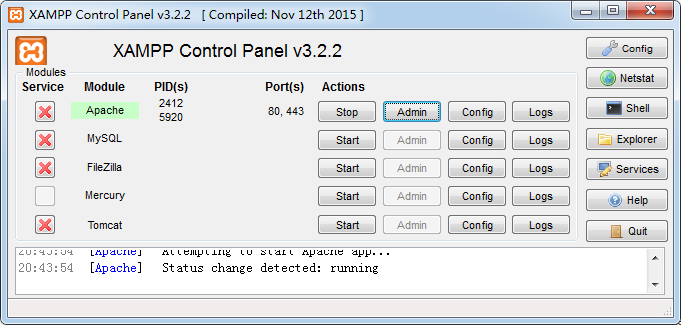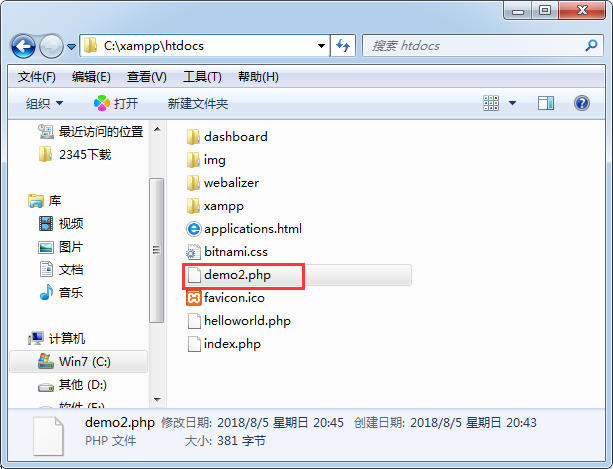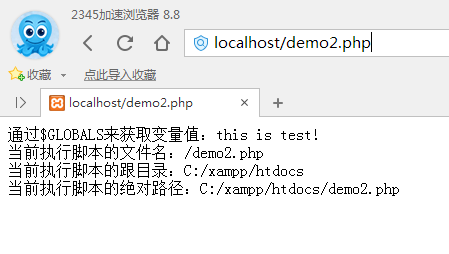# 常量

const name=value;

• __FILE__（放回当前文件名称，注意下划线是两个）
• __LINE__（返回当前代码所在行号，注意下划线是两个）
• __FUNCTION__（返回所在函数的名称，注意下划线是两个）
• __CLASS__（返回所在的类名，注意下划线是两个）
• PHP_OS（返回操作系统名称）
• TRUE（布尔值，真）
• FALSE（布尔值，假）
• NULL（空值）

demo3.php

<?php
echo "当前文件所在位置：".__FILE__."<br/>";
echo "当前操作系统是：".PHP_OS;

?>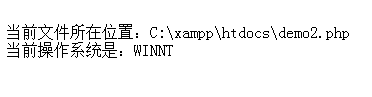# 赋值

demo4.php

<?php
$a="this is test!";//申明变量，并赋值$b;
$b='this is test!';//先申明变量，后赋值$c=$a;//使用一个变量给另一个变量赋值 ECHO$a."<br/>";
echo $b."<br/>"; echo$c."<br/>";

?>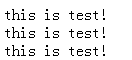## 字符串单引号和双引号

demo5.php

<?php
$a="this is test!";$b=10;
echo '$a'.'<br/>'.'$b'."<br/>";
echo "$a".'<br/>'."$b"."<br/>";

?>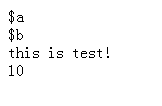# 语句结构

PHP语句通常是表达式组成，语句；（英文分号）结尾，不加会报错。

## 逻辑运算

• and/&&/&（逻辑与true&true==>true）
• or/||/|（逻辑或false||false==>false）
• not/!（逻辑非!true==>false）
• xor/^（逻辑异或，true^false==>true ，false^true==>true，false^false==>false）

### 逻辑与（&&）的短路原则：

• exp1&&exp2，如果exp1为false，则exp2不执行，直接返回false

## 分支结构

• if语句
• if-else语句
• if-elseif-else语句
• switch语句

demo6.php

<?php
$a=5;$b=10;
if($a>$b){
echo '$a大于$b';
}else{
echo '$a小于$b';
}

?>


## 循环结构

while语句

do-while语句

for语句

demo7.php

<?php
//for循环
for($i=0;$i<10;$i++){ echo 'good! '; } ?>  输出good! good! good! good! good! good! good! good! good! good! # 函数 PHP的函数很好用，不用申明返回数据的类型（当然这是优点也是缺点，不过我认为还是优点，减少了代码量啊，有没有！而且还不用想破头到底应该返回什么类型数据，而且PHP的输出支持链式调用，太方便了啊！） 函数把一个完整的功能多条语句封装成一个模块，函数使用function来申明 demo8.php <?php //输出n次变量a function printString($a,$n){ //for循环 for($i=0;$i<$n;$i++){ echo "$a";
}
}
//调用函数
printString("good! ",5);
printString("<br/>",2);
printString("apple and pear! ",1);

?>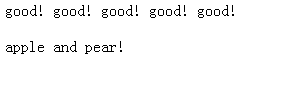这个时候的函数参数为值传递，就是一个copy，在函数内部改变这个值不会影响到外部的变量的值，但是如果我们想要在函数内部改变外部的值，需要引用传递，将参数的引用路径地址&a传入

demo.9.php

<?php
//交换$a,$b位置
function swap(&$a,&$b){
echo "交换前：".'$a='.$a.'$b='.$b;
echo '<br/>';
$temp=$a;
$a=$b;
$b=$temp;
echo "交换后：".'$a='.$a.'$b='.$b;
}
$a=5;$b=9;
//调用
swap($a,$b)

?>


交换前：$a=5$b=9



### 全局变量

<?php

$a=100; //输出全局变量a function gbl(){ global$a;
echo $a; } //调用 gbl(); ?>  输出 100  ### 静态变量 函数外不能直接使用函数内部的变量，如果需要在函数外使用申明的函数中的变量，函数中变量需要加前缀static <?php //输出静态变量a function stc(){ static$a=0;
$a++; echo$a.',';
}
//调用
stc();
stc();
stc();
stc();
stc();
?>


1,2,3,4,5,


### 返回值

PHP的函数不用声明返回值类型，真方便！

<?php
//返回字符型
function reutrnString(){
return "today is sunday.";
}
//返回数字
function returnNum(){
return 1024;
}
//调用
$a=reutrnString(); echo "\$a=$a"."<br/>";$a=returnNum();
echo "\$a=$a";

?>


$a=today is sunday.$a=1024

### 函数引用返回

（这个有点变态，直接操作引用地址，用起来出错了怎么搞）

<?php

//返回数字
function &returnNum(){
static $b=0;$b=$b+1; echo$b.'<br/>';
return $b; } //调用$a=returnNum();
//使用&
$a=&returnNum();$a=1000;
$a=returnNum(); ?>  输出 1 2 1001  ### 可变函数 需要在调用函数的时候在前面加$（申明变量符号），调用同一个函数，但是却执行的是不一样的代码，

（这个更加变态，不说了，看代码）

<?php
//返回字符型
function reutrnString(){
echo "today is sunday.<br/>";
}
//返回数字
function returnNum(){
echo 1024;
}
function hello(){
echo '你好';
}
//调用
$func='reutrnString';$func();
$func='returnNum';$func();
$func='hello';$func();

?>


today is sunday.
1024你好


### 递归函数

<?php
//递归
static $a=0;$a++;
echo '$a='.$a.",";
if($a>10){ return; } //调用自己，直到满足条件 add(); } add(); ?>  输出 $a=1,$a=2,$a=3,$a=4,$a=5,$a=6,$a=7,$a=8,$a=9,$a=10,$a=11,


### 系统函数

<?php
//返回最大值
$a=max(1,22,34,777,3,55); echo "1,22,34,777,3,55中最大的数是：".$a;
?>


1,22,34,777,3,55中最大的数是：777PHP基本语法
PHP基本语法总结
0 00 0php操作mysql防止sql注入(合集)
php操作mysql防止sql注入(合集)
0 0Centos中查看nginx、apache、php、mysql配置文件路径
Centos中查看nginx、apache、php、mysql配置文件路径
0 0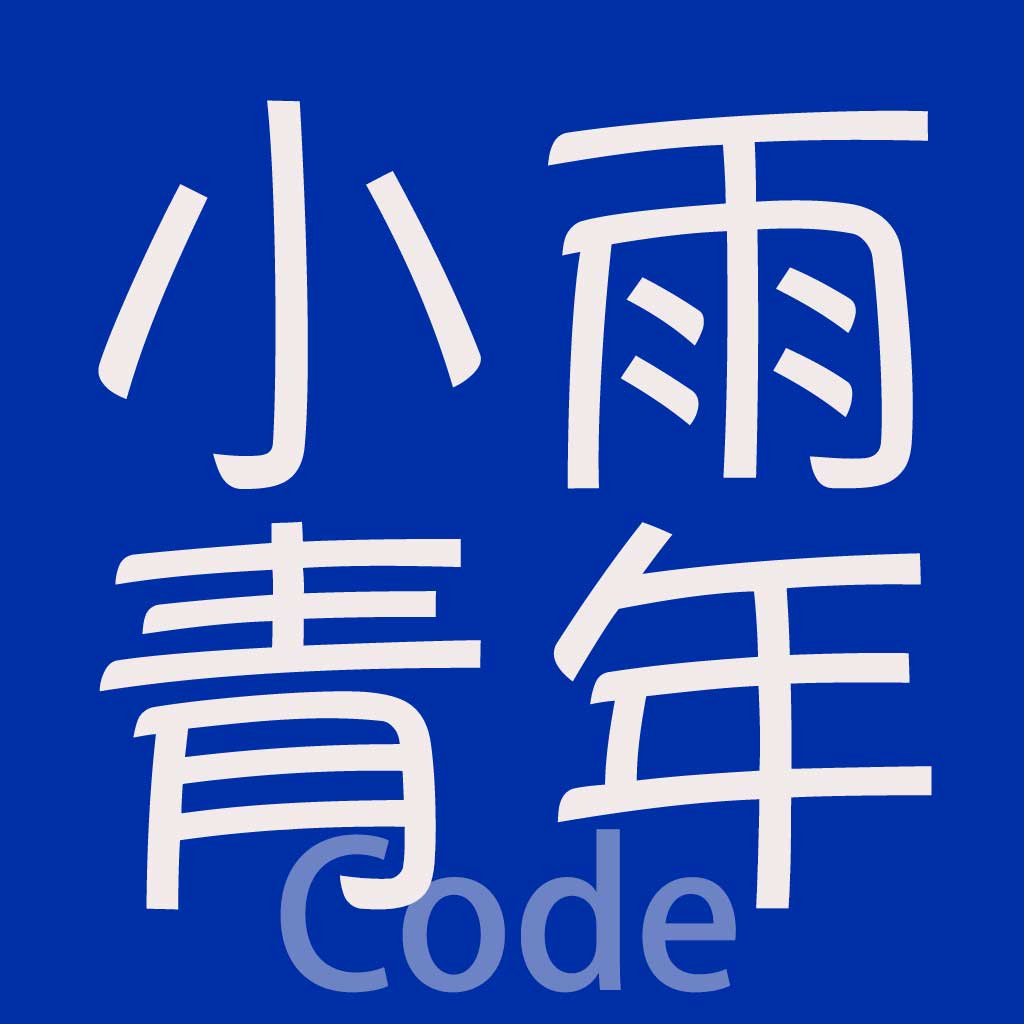MySQL事务的四种隔离类型以及PHP框架Yii2中的源码解读和实际应用
MySQL事务的四种隔离类型以及PHP框架Yii2中的源码解读和实际应用
0 00 0PHP连接MySQL 8.0报错的解决办法
PHP连接MySQL 8.0报错的解决办法
0 0PHP连接MySQL 8.0报错的解决办法
PHP连接MySQL 8.0报错的解决办法
0 0PHP+MySQL打造XXX管理系统
PHP+MySQL打造XXX管理系统
0 0PHP error：mysql_fetch_row() expects parameter 1 to be resource, boolean given 的错误
PHP error：mysql_fetch_row() expects parameter 1 to be resource, boolean given 的错误
0 0

PHP完全自学手册文档教程941088

PHP进阶教程 - 由浅入深掌握面向对象开发 - 第三阶段20931

PHP进阶教程 - 由浅入深掌握面向对象开发 - 第二阶段13933

PHP进阶教程 - 由浅入深掌握面向对象开发 - 第一阶段20432

PHP基础入门课程（上）38140

PHP基础入门课程（下）19842

PHP运行机制初探

CentOS Nginx PHP JAVA多语言镜像使用手册

CentOS Nginx PHP JAVA 多语言镜像使用手